# Deduction theorem

(diff) ← Older revision | Latest revision (diff) | Newer revision → (diff)

A general term for a number of theorems which allow one to establish that the implication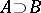can be proved if it is possible to deduce logically formulafrom formula. In the simplest case of classical, intuitionistic, etc., propositional calculus, a deduction theorem states the following: If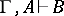(is deducible from the assumptions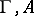), then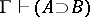(*)

(may be empty). In the presence of quantifiers an analogous statement is false:but not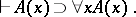One of the formulations of a deduction theorem for traditional (classical, intuitionistic, etc.) predicate calculus is: If, then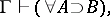whereis the result of prefixing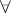quantifiers (cf. Quantifier) over all the free variables of the formula. In particular, ifis a closed formula, a deduction theorem assumes the form (*). This formulation of a deduction theorem makes it possible to reduce proof search in axiomatic theories to proof search in predicate calculus: Formulais deducible from axioms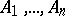if and only if the formulais deducible in predicate calculus.

A deduction theorem is formulated in a similar manner for logics with operations "resembling" quantifiers. Thus, a deduction theorem has the following form for the modal logics S4 and S5 (cf. Modal logic):More precise formulations of deduction theorems are obtained if one introduces-quantifiers not over all the free variables, but only over those bounded by quantifiers in the course of the deduction. One says that a variableis varied for a formulain a given deduction ifis free inand if the deduction under consideration involves an application of the rule of-introduction into the conclusion of an implication (or the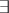-introduction into a premise), in which the quantifier overis introduced, and the premise of the application depends onin the proof under consideration. A deduction theorem for traditional predicate calculus may now be rendered more precise as follows: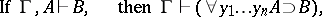where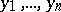is the complete list of variables varied forin the deduction under consideration. In particular, if no free variable inis varied, a deduction theorem assumes the form (*). In formulating the appropriate refinements of a deduction theorem for modal logics it should be borne in mind that the variation takes place in the rules for the introduction of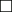into the conclusion of the implication andinto the premise.

In establishing a deduction theorem for the calculus of relevant implication (i.e. for systems adapted to the interpretation ofas "B is deducible with essential use of assumption A" ) one has the alternative of modifying the concept of the deduction itself or else of assuming that the variation takes place in any use of a "secondary" assumption; for instance, on passing from the pair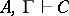,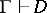to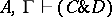, formulais varied, since it does not form part of the second member of the pair.

If, in a given deduction,is not varied, a deduction theorem assumes the form (*), and ifis varied it takes the following form:whereis a "true" constant (or the conjunction of the formulas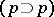for all propositional variablesin,,).

How to Cite This Entry:
Deduction theorem. Encyclopedia of Mathematics. URL: http://encyclopediaofmath.org/index.php?title=Deduction_theorem&oldid=17084
This article was adapted from an original article by G.E. Mints (originator), which appeared in Encyclopedia of Mathematics - ISBN 1402006098. See original article